Physical Chemistry : Orbitals and Hybridization

Example Questions

Example Question #1 : Orbitals And Hybridization

What is the hybridization on the nitrogen atom in a molecule of ammonia?

sp

sp2

sp3d

sp3

sp3

Explanation:

The hybridization of an atom can be determined by the number of atoms it is bonded to, as well as the number of lone pairs it has. Two of these variables would be sp, three variables would be sp2, and four would be sp3.

The nitrogen in ammonia is bonded to three atoms of hydrogen, but also has a lone pair in order to satisfy its octet. This means that nitrogen exhibits sphybridization.

Example Question #1 : Atoms And Elements

Which of the following are true regardingandorbitals?

I. Bothandorbitals can contain a maximum of two electrons

II. In a given shell,orbitals are more numerous because they are spherical in shape

III.orbitals have lower energy thanorbitals within the same shell

III only

I and II

I only

I and III

I and III

Explanation:

Orbitals are regions in an electron shell where electrons might be located. There are several types of orbitals such as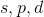, and. Most elements found on the periodic table contain electrons within one of these orbitals. A characteristic of an orbital is that it can only contain two electrons maximum. A shell might contain multiple orbitals; however, each orbital can only contain two electrons. Each orbital has a unique shape that corresponds to the electron density (the possible location of an electron at a given point in time). Theorbital has a spherical shape whereas theorbital has a dumbbell shape. As mentioned, a shell can contain multiple types of orbitals. A shell can typically contain oneorbital, threeorbitals, fiveorbitals, and sevenorbitals. Remember that the shape of the orbital has no bearing on the amount of orbitals in a shell. An orbital is higher in energy if it is found farther away from the nucleus. The orbitals in order of increasing energy is as follows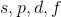. Therefore, anorbital has lower energy than aorbital in the same shell.

Example Question #43 : Nuclear, Quantum, And Molecular Chemistry

What is true when comparing the electron configuration of elemental sodium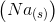and sodium cation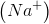?

The sodium ion has one additional electron in anorbital

The sodium ion has more electrons inorbitals

The outermost shell of the sodium ion has one electron

Elemental sodium is paramagnetic

Elemental sodium is paramagnetic

Explanation:

To answer this question, we need to find the electron configuration of both elemental sodium and sodium cation. If we look at the periodic table we can see that sodium is found on the first column. Since it is found in the first column, sodium has one valence electron. To complete octet, sodium will readily lose an electron and become a positively charged sodium ion. The electron configuration for sodium is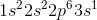. The electron configuration for sodium ion is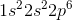(because it lost its electron in the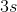orbital). This means that elemental sodium has an unpaired electron in itsorbital; the sodium ion has no unpaired electrons. Recall that an unpaired electron can generate its own magnetic field and is called paramagnetic; therefore, solid sodium is paramagnetic. The number of electrons in theorbitals for both sodium and sodium ion is the same (6 electrons total in the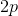orbital). The outermost shell of sodium is the third shell (because sodium is located on the third row of periodic table). Elemental sodium contains one electron in theorbital in the outermost shell whereas the sodium ion contains 6 electrons in its outermost shell.

Example Question #44 : Nuclear, Quantum, And Molecular Chemistry

It is observed that a molecule has three hybridized orbitals in its outermost shell. What can you conclude about this molecule?

None of these

It has a lone pair electron

It has a double bond

It has four single bonds

It has a double bond

Explanation:

Hybridization is a process involving the fusion, or hybridization, ofandorbitals to form a unique orbital. It is possible for various combinations ofandhybridization. Recall that there is oneorbital and threeorbitals in each shell. This means that the oneorbital can hybridize with 1, 2, or all 3orbitals. Since there are three total combinations, there are three types of hybridized orbitals. These are,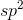, and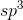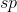orbital has oneand oneorbital hybridized. This means that theorbital and the firstorbital become a neworbital. A molecule withhybridization will have twoorbitals and twoorbitals. Similarly, anorbital is made from the hybridization of oneand twoorbitals. Inhybridization, there are threeorbitals and oneorbital. Finally, anorbital has oneand all threeorbitals; therefore, anhybridized molecule will have fourorbitals and noorbitals. The question states that there are three hybridized orbitals in this molecule; therefore, this molecule must behybridized. The singleorbital is unhybridized because the molecule probably has a double bond. Electrons inbonds in double and triple bonds cannot be found in hybridized orbitals; therefore, they need their ownorbital. If a molecule has onebond (double bond), then it will need oneorbital and will behybridized (because this will give threehybridized orbitals and oneorbital). If it has twobonds (triple bond), then it will need twoorbitals and will behybridized. If a molecule has all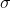bonds (single bonds), then the molecule will require no emptyorbitals for the delocalized electrons, and will behybridized.

Example Question #2 : Orbitals And Hybridization

Which of the following is true regarding carbon tetrachloride?

Hybridization in this molecule involves three times as manyorbitals asorbitals

Hybridization in this molecule involves two times as manyorbitals asorbitals

More than one of these are true

The carbon in this molecule has similar hybridization as the carbon in carbon dioxide

Hybridization in this molecule involves three times as manyorbitals asorbitals
Carbon tetrachloride,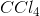, has a central carbon atom attached to four chlorine atoms. The bonds between the carbon atom and chlorine atoms are single covalent bonds. The electrons in a single bond (bond) can be found in hybridized orbitals. Since carbon tetrachloride only has single bonds, the carbon atom can hybridize all of its orbitals (oneand three) in the outermost shell and form ahybridization; therefore, threeorbitals and oneorbital participate in hybridization leading us to the correct answer. Carbon dioxide,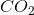, has a central carbon atom bonded to two oxygen atoms. To complete octet, carbon and oxygen atoms have double bonds. This means that carbon dioxide has twobonds (two double bonds). Recall that electrons inbonds cannot reside in hybridized orbitals; therefore, to accommodate the twobonds we need two empty, unhybridizedorbitals. This means that carbon dioxide will have hybridization of oneand oneorbital, giving it anhybridization.